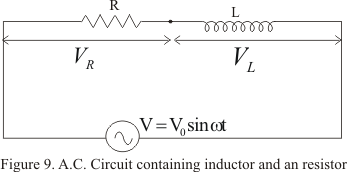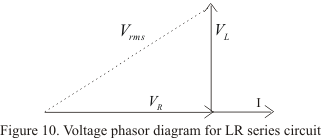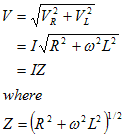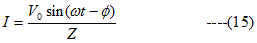# Circuit containing inductance and resistance in series|Alternating Current

## Circuit containing inductance and resistance in series

• Figure below shows pure inductor of inductance L connected in series with a resistor of resistance R through sinusoidal voltage
V=V0sin(ωt+φ)• An alternating current I flowing in the circuit gives rise to voltage drop VR across the resistor and voltage drop VL across the coil
• Voltage drop VR across R would be in phase with current but voltage drop across the inductor will lead the current by a phase factor π/2
• Now voltage drop across the resistor R is
VR=IR
and across inductor
VL=I(ωL)
where I is the value of current in the circuit at a given instant of time
• So voltage phasors diagram isIn figure (10) we have taken current as a reference quantity because same amount of current flows through both the components. Thus fro phasors diagramis known as impedance of the circuit
• Current in steady state isand it lags behind applied voltage by an angle φ such that
tanφ=ωL/R                                                      ---(16)

Latest Articles
Synthetic Fibres and Plastics Class 8 Practice questions

Class 8 science chapter 5 extra questions and Answers

Mass Calculator

3 Fraction calculator

Garbage in Garbage out Extra Questions7